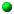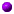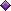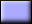# Repetitive Execution### Select the topics you wish to review:Repetitive ExecutionCounting DO-LoopProgramming Examples:Counting Positive and Negative Input ValuesComputing Arithmetic, Geometric and Harmonic MeansComputing FactorialGeneral DO-Loop with EXITProgramming Examples:Determining the Minimum and Maximum of Input DataComputing the Square Root of a Positive NumberComputing EXP(x)Computing the Greatest Common Divisor of Two Positive IntegersChecking If a Positive Integer Is a Prime NumberNested DO-LoopProgramming Examples:Computing Classes AveragesComputing a Set of Values of EXP(x)Armstrong NumbersFinding All Primes in the Range of 2 and NFinding all Prime factors of a Positive IntegerHandling End of File: the READ Statement RevisitedComputing Arithmetic, Geometric and Harmonic Means: RevisitedThe DO-CYCLE Construct and a Programming Example (Optional)Download my course overheads